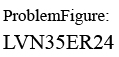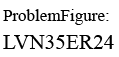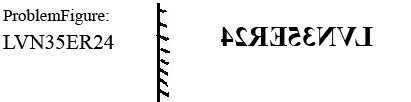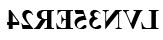Question 1

# Which of the option figures is the exact mirror image of the given problem figure when the mirror is held to the right side of the problem figure?Solution

Given figurethe above figure mirror image is given below  held to the right side of the problem figurethen  the mirror image istherefore Option (C) Ans

• Free SSC Study Material - 18000 Questions
• 230+ SSC previous papers with solutions PDF